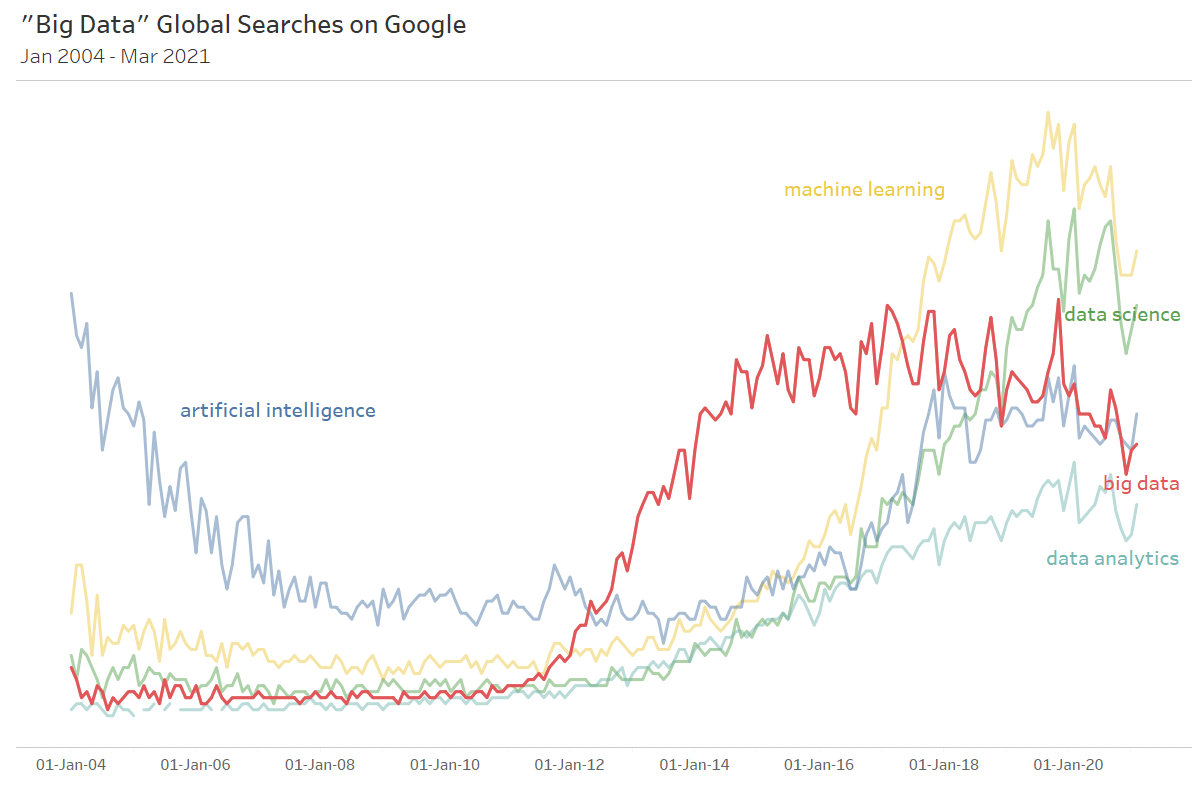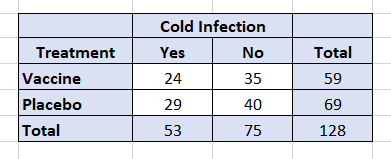“Big data.”

We checked in with Google search trends recently. Appears that “Big Data” has lost its luster search-wise…started trending down about 4 years ago.## Implications of big data

However, this does not mean we should lose sight of certain statistical implications associated with being “big”. Yes, large amounts of data can help us estimate relationships (effects) with a high degree of precision.

And help us uncover low occurrence events such as the blood clotting cases associated with the Johnson & Johnson COVID-19 vaccine.

But massive amounts of data can reveal patterns that are not always meaningful or happen by chance.

Additionally, from a statistical inference perspective, with big data, even small, uninteresting effects can be statistically significant.

This has important implications for inferential conclusions about the associations we are studying.

And it does not take all that much data for this to happen.

### Small clinical trial example

As an example, consider the following hypothetical results from a clinical trial of a “common” cold vaccine:The table shows the number of subjects who had both a positive outcome (no infection) and negative outcome (infection) across the two types of treatment. A standard statistical test of association, the Pearson chi-squared, indicates we cannot say there is any difference in outcomes across the two treatment types.

That is, we cannot reject the “null” hypothesis of no association at the 95% level of confidence (i.e., X2 = 0.024).

The strength of the association, or effect size, is obtained from the ratio of relative risks.

The probability of a vaccinated subject getting sick is (24 / 59) or 0.407 (40.7%) while that for the placebo group is (29 / 69) or 0.420 (42.0%).

So the relative risk ratio is (0.407 / 0.420) or 0.968.

Thus, we would expect that when applied to the population, under the same conditions as the study, there would be 3.2% fewer infections among those who received the vaccine (i.e., (1 – 0.968) *100)).

This 3.2% is known as the efficacy rate of the vaccine.

The 95% confidence interval for the relative risk ratio is wide (i.e., 0.639 to 1.465) indicating a lack of precision in the point estimate of 0.968.

The study investigators conclude that the effect of the vaccine is neither statistically nor practically significant.

Aside from its statistical insignificance, an efficacy rate of just 3.2% is not nearly large enough to justify starting production of the vaccine.

### Large clinical trial example

Contrast this with the following study results based on a much larger sample of 44,800 subjects:The Pearson chi-squared statistic (X2) is now 8.375. Thus, the hypothesis of no association can be rejected at the 95% level of confidence.

And the 95% confidence interval for the relative risk ratio is much narrower indicating a much higher level of precision (i.e., 0.947 to 0.990).

The study investigators now conclude that there is a statistically significant association between receiving the vaccine and avoiding a cold infection (positive outcome).

But, the relative risk ratio of a positive outcome from receiving the vaccine is identical to that obtained from the smaller study, 0.968.

Implying the efficacy rate is also the same, 3.2%.

## Practical vs statistical significance

What are we to make of this?

From the perspective of effect size, do the larger study results carry more weight simply because the hypothesis of no association can be rejected? Even though the practical significance has remained the same?

We can turn a very small, 3.2% effect into a statistically significant effect by simply increasing the sample size.

But does this change the practical significance of the 3.2%?

No.

If 3.2% was deemed by the study investigators to be practically insignificant, it remains practically insignificant. Despite the larger sample size and despite it now being statistically significant.

## A curse of data “bigness”

With a large enough sample, everything is statistically significant, even associations that are practically not significant or very interesting.

The implication is that rather than focusing on hypothesis testing as sample sizes increase, the focus should shift. Towards the size of the estimated effect, whether the estimated effect is “practically” important, and “sensitivity analysis” (i.e., how does the estimated effect change when control variables are added and dropped).

Confidence intervals can and should play a role. But they will get narrower and narrower as sample sizes grow. And everything within the confidence interval could still be deemed not practically important.

In sum, as data get bigger (and it does not take massive amounts of data for this to be an issue), we need to guard against concluding that a small effect is practically significant just because the p-value is very small (i.e., the effect is statistically significant).

The curse of big data is still very much with us.

 A ratio of 1.0 would mean no difference in effect between the treatment types.

 As a point of comparison, the 2020 Moderna and Pfizer COVID-19 vaccines trials consisted of about 30,000 and 40,000 subjects.

 A more complicated technique is used to calculate confidence intervals for actual clinical trial results than used here, which typically result in wider intervals.  For example, in 2020, Moderna reported an efficacy rate of 94.1% for its COVID-19 vaccine with a 95% confidence interval of 89.3% to 96.8%.

 Since the standard error of the relative risk ratio estimate is based on the cell counts in the contingency table, increasing the size of the sample lowers the standard error, making it more likely we can reject the null hypothesis at a given level of confidence.

 The paper Too Big to Fail presents a nice discussion of these issues. Additionally, the American Statistical Association released recommendations on the reporting of p-values.

ost content here…#### Written by Kevin Duffy-Deno

Dr. Kevin Duffy-Deno, with over 30 years of experience in quantitative research and analysis, is an expert in econometric, data, statistical and predictive modeling and data visualization. Both with Bintel and prior organizations, Dr. Duffy-Deno has been engaged in numerous projects requiring data acquisition from proprietary and public sources, the normalization of such data and the integration of data into a format supporting analytic analyses and products.